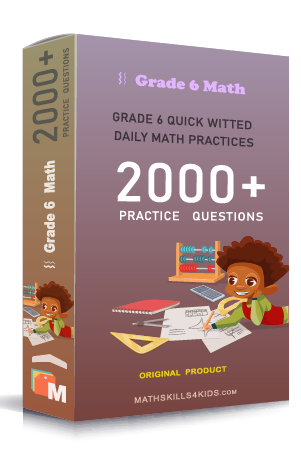# Grade 6 Number theory worksheets with solutions

Subject
Math

Resource Type
Worksheets, Printables, Homeschool

Standards
CCSS 6.NS.B.4

Format
PDF (26.7 MB | 52 pages → Exercises + Solutions)

• ### -15% OFF Over \$100

Promo Code: TOPLA

After Purchase

\$4.25

This package allows you to practice the following skills

Converting between standard and scientific notation

Comparing numbers written in scientific notation

Identifying prime and composite numbers

Identifying factors and multiples

Understanding prime factorization

Using a number tree to find prime factors

Writing prime factorization with exponents

Finding the GCF (greatest common factor) of numbers

Finding the LCM (least common multiple) of numbers

Solving GCF and LCM word problems

Sorting factors of numerical expressions

Classifying numbers :
whole numbers, fraction, rational numbers, integers

This product is a part of the Mega Pack
* Grade 6 quick-witted daily math practices *